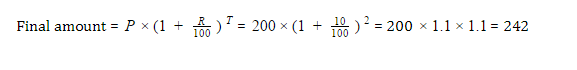# GMAT Quant: Simple & Compound Interest ProblemsGMAT Quant is not complete without Interest related questions. Interest is a portion of amount, generally given by the borrower to a lender. If you borrow money from someone or bank, you have to pay some extra money, as a return to a favour done by the lender. The borrower or lender could be a person, bank or a company.

In GMAT, two types of interest related questions are asked: Simple Interest and Compound Interest.

## Simple Interest

$\large Simple \; Interest = \frac{PRT}{100}$ $\large New \; Principle \; Amount = P + SI$

## Compound Interest

$\large Compound \; Interest \; (CI)= P \times \left ( 1 + \frac{R}{100} \right )^{T} – P$

Where,

P – Principle amount that has been borrowed
R – Rate of interest charged to the borrower
T – Time span for which the borrower has borrowed money
SI – Simple interest to be given
CI – Compound interest to be given.Let’s look at some of the Mathematical problems related to Simple and Compound Interest:

1. What is time in which a sum of Rs. 900 will yield Rs. 81 at the rate 0f 4.5% er annum if simple interest is taken?

SI = 81, P = 900 R = 4.5. Putting into the formula

81 = 900*4.5*T/100

T = 81/40.5 = 2 years

2. What will be the amount of Rs. 200 be after 2 years if the interest rate of 10% is compounded annually?We’ll be glad to help you in your GMAT preparation journey. You can ask for any assistance related to GMAT and MBA from us by calling us at +918884544444. You can write to us at gmat@byjus.com.